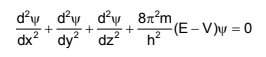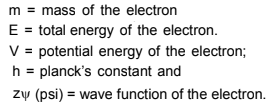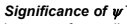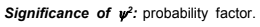# Quantum Mechanical Model of atom

The atomic model which is based on the particle and wave nature of the electron is known as wave or quantum mechanical model of the atom. This was developed by Ervin Schrodinger in 1926. This model describes the electron as a three dimensioinal wave in the electronic field of positively charged nucleus. Schrodinger derived an equation which describes wave motion of an electron. The differential equation iswhere x, y, z are certain coordinates of the electron,The wave function may be regarded as the amplitude function expressed in terms of coordinates x, y and z. The wave function may have positive or negative values depending upon the value of coordinates. The main aim of Schrodinger equation is to give solution for probability approach. When the equation is solved, it is observed that for some regions of space the value of  is negative. But the probability must be always positive and cannot be negative, it is thus, proper to useIt describes the probability of finding an electron within a small space. The space in which there is maximum probability of finding an electron is termed as orbital. The important point of the solution of the wave equation is that it provides a set of numbers called quantum numbers which describe energies of the electron in atoms, information about the shapes and orientations of the most probable distribution of electrons around nucleus.

Post By : Ramashankar Rajppot 19 Jan, 2020 2621 views Chemistry